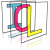Image Component Library (ICL)
icl::math::PolynomialRegression< T > Class Template Reference

Generic Implementation of the Polynomial Regression algorithm. More...

`#include <PolynomialRegression.h>`

## Classes

class  Result
result type More...

## Public Types

typedef DynMatrix< T > Matrix
internally used matrxi type More...

typedef PolynomialRegressionAttrib< T > Attrib
internally used type More...

## Public Member Functions

PolynomialRegression (const std::string &function)
create instance with given function generator More...

const Resultapply (const Matrix &xs, const Matrix &ys, bool useSVD=false)
computes the polynomial regression parameters More...

std::string getFunctionString () const
returns the interpreted function string More...

## Protected Attributes

Matrix m_buf
internal buffer More...

Result m_result
internal result buffer (always returned as const-reference) More...

## Detailed Description

### template<class T> class icl::math::PolynomialRegression< T >

Generic Implementation of the Polynomial Regression algorithm.

Polynomial regression applies least square regression based on a polynomial that is computed on the input variables. It minimized p(x) a = y, for a given polynomial function p(x). x can be a scalar of a vector. The same is true for y. Each entry of x and y resp. is assued to be one row of the input matrices xs and ys.

# Result

The resulting PolynomialRegression::Result instance can be used to compute the output of the a given set of input variables simultaneously. It contains the optimal solution of a, which is used to compute y = p(x) a.

# The Polynomial Function definition

The PolynomialRegression class uses a rather very simple but intuitive syntax for the definition of the used polynomial. Here is an example that contains all allowed parts: "1 + x0 + x1 + x0*x1 + x1^2 + x2^2" White spaces are ignored; tokens are defined by the "+"-delimiter. Tokens can be of form

• K, where K is a constant (can be float, however actually only "1" makes sence here)
• xI, where I is an integer input variable index
• xI*xJ*... , where I and J and other are integer input varialbe indices
• xI^K, where K is a constant (can be float and int)

## ◆ Attrib

template<class T >
 typedef PolynomialRegressionAttrib icl::math::PolynomialRegression< T >::Attrib

internally used type

## ◆ Matrix

template<class T >
 typedef DynMatrix icl::math::PolynomialRegression< T >::Matrix

internally used matrxi type

## ◆ PolynomialRegression()

template<class T >
 icl::math::PolynomialRegression< T >::PolynomialRegression ( const std::string & function )

create instance with given function generator

## ◆ apply()

template<class T >
 const Result& icl::math::PolynomialRegression< T >::apply ( const Matrix & xs, const Matrix & ys, bool useSVD = `false` )

computes the polynomial regression parameters

The input matrices xs and ys must have the same number of rows, and xs needs to have at least m_attribMaxIndex+1 columns. The input dimension is given by the number of columns in xs, the output dimension is defined by the number of columns in ys. Each row of xs and ys resp. defines a single input/output pair that is used for the internal least-square based optimization

Optionally, the internally computed pseudo inverse that solves p(xs) a = ys, which is p(xs).pinv() can be computed using an SVD-based approach. This is usually slower, but more stable and less prone to singular-matrix-exceptions.

## ◆ getFunctionString()

template<class T >
 std::string icl::math::PolynomialRegression< T >::getFunctionString ( ) const

returns the interpreted function string

## ◆ m_buf

template<class T >
 Matrix icl::math::PolynomialRegression< T >::m_buf
mutableprotected

internal buffer

## ◆ m_result

template<class T >
 Result icl::math::PolynomialRegression< T >::m_result
protected

internal result buffer (always returned as const-reference)

The documentation for this class was generated from the following file: